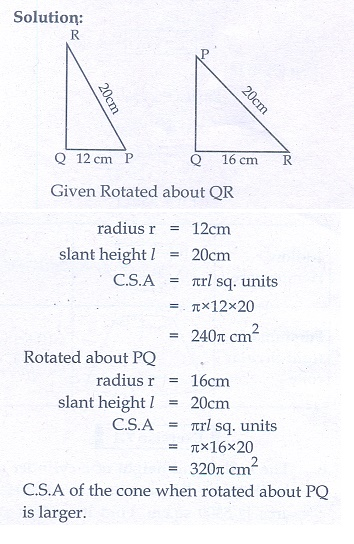Home | | Maths 10th Std | Exercise 7.1: Surface Area

# Exercise 7.1: Surface Area

Surface Area of Right Circular Cylinder, Surface Area of Hollow Cylinder, Surface Area of Right Circular Cone, Surface Area of The Sphere, Surface Area: Frustum of a right circular cone. (Maths Book back answers and solution for Exercise questions)

Exercise 7.1

1. The radius and height of a cylinder are in the ratio 5:7 and its curved surface area is 5500 sq.cm. Find its radius and height.2. A solid iron cylinder has total surface area of 1848 sq.m. Its curved surface area is five – sixth of its total surface area. Find the radius and height of the iron cylinder.3. The external radius and the length of a hollow wooden log are 16 cm and 13 cm respectively. If its thickness is 4 cm then find its T.S.A.4. A right angled triangle PQR where Q = 90 is rotated about QR and PQ. If QR=16 cm and PR=20 cm, compare the curved surface areas of the right circular cones so formed by the triangle.5. 4 persons live in a conical tent whose slant height is 19 cm. If each person require 22 cm2 of the floor area, then find the height of the tent.6. A girl wishes to prepare birthday caps in the form of right circular cones for her birthday party, using a sheet of paper whose area is 5720 cm2, how many caps can be made with radius 5 cm and height 12 cm.7. The ratio of the radii of two right circular cones of same height is 1:3. Find the ratio of their curved surface area when the height of each cone is 3 times the radius of the smaller cone.8. The radius of a sphere increases by 25%. Find the percentage increase in its surface area.9. The internal and external diameters of a hollow hemispherical vessel are 20 cm and 28 cm respectively. Find the cost to paint the vessel all over at ₹ 0.14 per cm2 .10. The frustum shaped outer portion of the table lamp has to be painted including the top part. Find the total cost of painting the lamp if the cost of painting 1 sq.cm is ₹ 2.1. 25 cm, 35 cm

2. 7 m, 35 m

3. 2992 sq.cm

4. CSA of the cone when rotated about PQ is larger.

5. 18.25 cm

6. 28 caps

7. √5 : 9

8. 56.25%

9. ₹ 302.72

10. ₹ 1357.72

Tags : Problem Questions with Answer, Solution | Mensuration | Mathematics , 10th Mathematics : UNIT 7 : Mensuration
Study Material, Lecturing Notes, Assignment, Reference, Wiki description explanation, brief detail
10th Mathematics : UNIT 7 : Mensuration : Exercise 7.1: Surface Area | Problem Questions with Answer, Solution | Mensuration | Mathematics